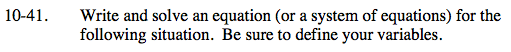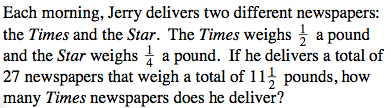### Home > AC > Chapter 10 > Lesson 10.1.4 > Problem10-41

10-41.$t + s = 27 \ \ \ \ \ \ \ \ \ t = \# \text{ of Times}$

$\frac{1}{2}t + \frac{1}{4}s = 11\frac{1}{2} \ \ \ s = \# \text{ of Stars}$

Multiply the bottom equation by − 4 to remove the fractions.
t + s = 27
− 2ts = − 46
t = − 19
t = 19